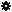﻿ Object Model: VertexCollection.NeighborPolygons

VertexCollection.NeighborPolygons

## VertexCollection.NeighborPolygonsv3.0

### Description

Returns a collection PolygonFace objects for all polygon neighbors within the specified distance.

### C# Syntax

 ```PolygonFaceCollection VertexCollection.NeighborPolygons( Int32 in_lDistance ); ```

### Scripting Syntax

 ```oReturn = VertexCollection.NeighborPolygons( [Distance] ); ```

### Parameters

Parameter Type Description
Distance Integer Distance is an integer value representing the degree of neighborhood (eg: degree=2 for a vertex means its adjacent polygons plus the adjacent polygons of the adjacent polygons)

Default Value: 1

### Examples

#### VBScript Example

 ```set oCube = ActiveSceneRoot.AddGeometry( "Cube", "MeshSurface" ) set oGeometry = oCube.ActivePrimitive.Geometry set oPolygons = oGeometry.Polygons set oNeighborVertices = oPolygons(0).NeighborVertices(1) set oNeighbors = oNeighborVertices.NeighborPolygons str = "Polygons neighbors of this VertexCollection are :" for each n in oNeighbors str = str & " " & n.index next logmessage str ```